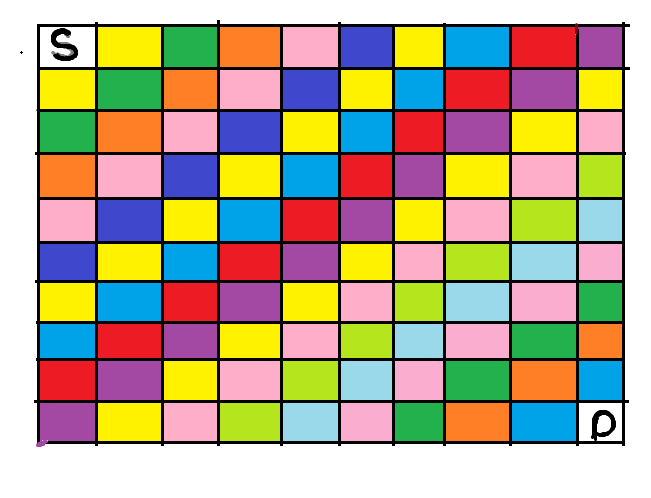# Can You reach the end | RECHEND | Oct-2021-cook off | Explanation with diagram

QueLink : Contest Page | CodeChef

Explanation: As in question it is clearly mentioned that in each row and in each column there is only a single block, (cell which we cannot cross) , so now how many different scenarios are possible,

we cannot reach our destination if we have all blocks arranged (as in question says every row or column contain a single block) , on a diagonal.

In the Below image we can clearly see that how many different diagonals can be possible, each diagonal colored with the same color.

So just each diagonal coordinate present in given blocks, if yes that means we cannot reach our destination, means that diagonal will block our whole path to reach the destination.``````lli tt =1;
cin>>tt;
while(tt--)
{
lli n;
cin>>n;
set<pair<int,int>>st;
loop(i,n)
{
lli x,y;
cin>>x>>y;
st.insert({x,y});
}
bool f = false;
for(int i=1;i<=n;i++)
{
bool flag=false;
lli row = i;
lli col = 1;
while(row>=1)
{
if(st.find({row,col})==st.end())
{
flag=true;
break;
}
row--;
col++;
}

if(flag==false){
f=true;
break;
}
}

for(int j=1;j<n;j++)
{
bool flag=false;
lli row = n;
lli col = j;
while(col<=n)
{
if(st.find({row,col})==st.end())
{
flag=true;
break;
}
row--;
col++;
}

if(flag==false){
f=true;
break;
}
}

if(f)
print("NO");
else
print("YES");
}
``````

3 Likes

void solve()
{
int t;cin>>t;
while(t–)
{
ll n,a,b;cin>>n;bool ans=0;int pos1,pos2,pos3,pos4;
map<int,int> Mp;
fo(i,0,n)
{
cin>>a>>b;

``````  Mp[a+b-2]++;
``````

}
debug(Mp);
debug(pos1);
debug(pos2);

rep(Mp)
{
if(it->ff!=0 && it->ff!=2*(n-1))
{
if(it->ff<=n-1)
{
if(it->ss==it->ff+1)
{
cout<<“NO”<<nline;
ans=1;
break;
}
}
else
{
if(it->ss==(((2*(n-1))-(it->ff))+1))
{
cout<<“NO”<<nline;
ans=1;
break;
}
}
}
}
if(ans==0)
{
cout<<“YES”<<nline;
}

}

}